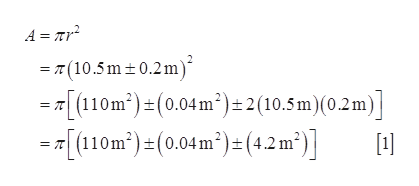# The radius of a circle is measured to be (10.5 ± 0.2) m. Calculate(a) the area and (b) the circumference of the circle, andgive the uncertainty in each value.

Question
8 views

The radius of a circle is measured to be (10.5 ± 0.2) m. Calculate
(a) the area and (b) the circumference of the circle, and
give the uncertainty in each value.

check_circle

Step 1

(a) The area of the circle ishelp_outlineImage TranscriptioncloseA = Tr? = T (10.5 m±0.2m)´ -=[(110m²)=(0.04m²)±2(10.5 m)(0.2m)] =[(110m²) =(0.04m²)= (4.2 m²)]  1.21 fullscreen
Step 2

Neglect the middle term in equation (1) as it is smaller value com...

### Want to see the full answer?

See Solution

#### Want to see this answer and more?

Solutions are written by subject experts who are available 24/7. Questions are typically answered within 1 hour.*

See Solution
*Response times may vary by subject and question.
Tagged in

### Other